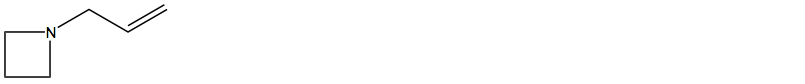# Problem: Determine the molecular formula corresponding to the following structure. Calculate the number of degrees of unsaturation from the molecular formula and evaluate whether your calculations agree with the structure shown.

###### Problem Details

Determine the molecular formula corresponding to the following structure. Calculate the number of degrees of unsaturation from the molecular formula and evaluate whether your calculations agree with the structure shown.